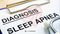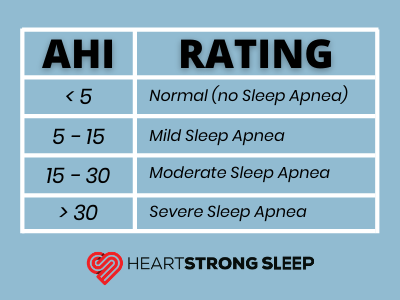Apnea-Hypopnea Index (AHI) measures sleep apnea severity. The AHI is the sum of the number of apneas (pauses in breathing) plus the number of hypopneas (periods of shallow breathing) that occur, on average, each hour.

To count in the index apneas and hypopneas, collectively called events, must have a duration of at least 10 seconds.

The AHI, as with the separate Apnea Index and Hypopnea Index, is calculated by dividing the number of events by the number of hours of sleep.From the AHI rating chart here, we see that an index less that 5 is considered normal. For an Apnea-Hypopnea Index (or AHI) from 5 to 15 denotes mild sleep apnea. Fifteen to 30 is moderate, while a greater than 30 AHI is considered severe.

• AHI = sum of the number of apneas (pauses in breathing) plus the number of hypopneas (periods of shallow breathing) occurring, on average, each hour.
• Apneas and hypopneas, collectively called events, must last at least 10 seconds to count as events.
• The AHI is calculated by dividing the number of events by the number of hours of sleep.

In summary, if you are snoring or someone tells you that you do, we'd recommend a home sleep test.

Sleep apnea